# PSAT Math : How to find the perimeter of a square

## Example Questions

### Example Question #1 : How To Find The Perimeter Of A Square

A circle with a radius 2 in is inscribed in a square. What is the perimeter of the square?

16 in

24 in

28 in

12 in

32 in

16 in

Explanation:

To inscribe means to draw inside a figure so as to touch in as many places as possible without overlapping. The circle is inside the square such that the diameter of the circle is the same as the side of the square, so the side is actually 4 in.  The perimeter of the square = 4s = 4 * 4 = 16 in.

### Example Question #11 : Quadrilaterals

Square X has 3 times the area of Square Y.  If the perimeter of Square Y is 24 ft, what is the area of Square X, in sq ft?

108

112

144

72

54

108

Explanation:

Find the area of Square Y, then calculate the area of Square X.

If the perimeter of Square Y is 24, then each side is 24/4, or 6.

A = 6 * 6 = 36 sq ft, for Square Y

If Square X has 3 times the area, then 3 * 36 = 108 sq ft.

### Example Question #1 : How To Find The Perimeter Of A Square

A square has an area of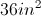.  If the side of the square is reduced by a factor of two, what is the perimeter of the new square?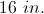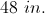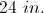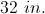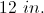The area of the given square is given by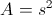so the side must be 6 in.  The side is reduced by a factor of two, so the new side is 3 in.  The perimeter of the new square is given by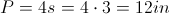.